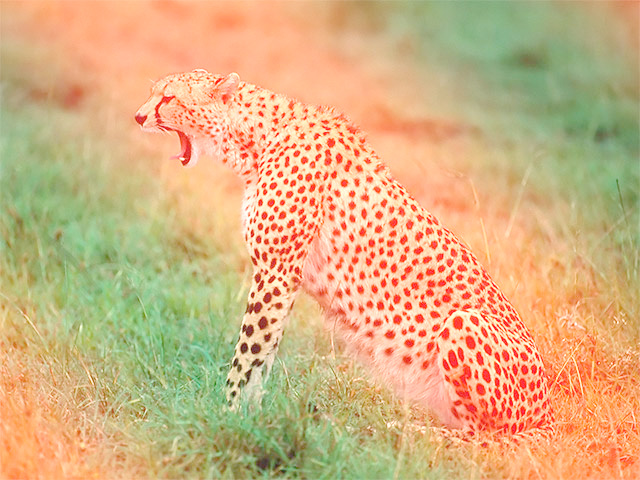Since version 0.3.36 OPENRNDR comes with compute shader functionality for select platforms. Compute shader support only works on systems that support OpenGL 4.3 or higher. This excludes all versions of MacOS.

## Example use

This example is composed of two code blocks. The first block is a compute shader program written in GLSL which produces an `outputImg` by mixing three input colors:

• A pixel sampled from `inputImg`.
• A `fillColor` sent as a uniform from Kotlin.
• A color generated by calculating the cosine of the coordinates of the pixel currently being processed.

A typical location for such a compute shader could be `data/compute-shaders/fill.cs`.

``````#version 430
layout(local_size_x = 1, local_size_y = 1) in;

uniform vec4 fillColor;
uniform float seconds;
uniform writeonly image2D outputImg;

void main() {
ivec2 coords = ivec2(gl_GlobalInvocationID.xy);
float v = cos(coords.x * 0.01 + coords.y * 0.01 + seconds) * 0.5 + 0.5;
vec4 wave = vec4(v, 0.0, 0.0, 1.0);

imageStore(outputImg, coords, wave + inputImagePixel + fillColor);
}
``````The second code block is an OPENRNDR program making use of the compute shader:

1. It creates a compute shader program from a file.
2. Initializes the input buffer using a image loaded from disk.
3. Inside the `extend` block (called multiple times per second) some uniforms are updated: a `fillColor`, the time in `seconds`, the `inputImg` and the `outputImg`. In this example only the time changes on every frame.
4. The compute shader is executed, writing the result to the `outputBuffer`.
5. Finally, the result is displayed by calling `drawer.image()`.
``````fun main() = application {
program {

val inputBuffer = colorBuffer(width, height)
tempBuffer.copyTo(inputBuffer)
val outputBuffer = colorBuffer(width, height)

extend {